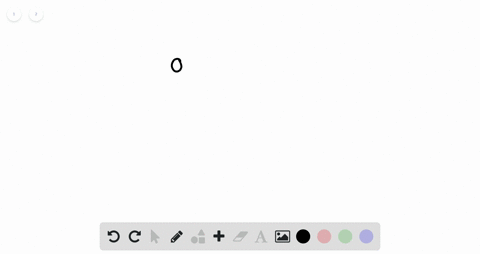Enroll in one of our FREE online STEM summer camps. Space is limited so join now!View Summer Courses### For Exercises $1-12,$ determine whether the order…

00:50California University of Pennsylvania

Need more help? Fill out this quick form to get professional live tutoring.

Get live tutoring
Problem 4

For Exercises $1-12,$ determine whether the ordered pair is a solution for the equation.
$$(4,-6) ; y=3 x-1$$

## Discussion

You must be signed in to discuss.

## Video Transcript

in order to determine if the 0.4 negative six is a solution to the equation. Y equals three X minus one. We're going to plug negative six into why and four into acts. So get negative. Six equals three times four minus one. So they have negative. Six equals 12 minus one negative. Six equals 11. Since negative six does not equal 11 the 110.4 negative six is not a solution.